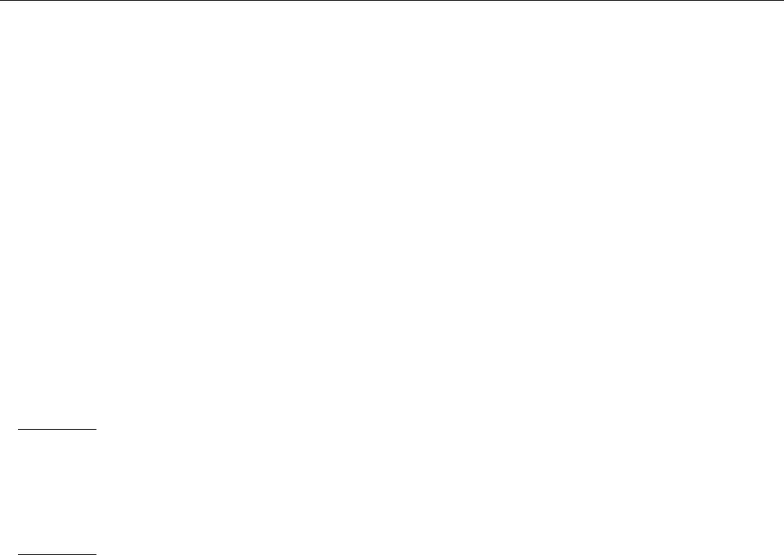Class Notes (1,100,000)
CA (620,000)
McGill (30,000)
COMP (700)
Lecture 2

# COMP 273 Lecture Notes - Lecture 2: Normalized Number, Single-Precision Floating-Point Format, Decimal Mark

Department
Computer Science (Sci)
Course Code
COMP 273
Professor
Piotr Przytycki
Lecture
2

This preview shows pages 1-2. to view the full 6 pages of the document.COMP 273 2 - IEEE ﬂoating point Jan. 13, 2016
Fixed Point
We are mostly discussing ﬂoating point numbers today, but I want to mention brieﬂy that some
computers represent non-integers numbers using ﬁxed point. Fixed point means we have a constant
number of bits (or digits) to the left and right of the binary (or decimal) point.
For example, we might have eight digits to the left of the decimal point and two digits to the
right. An example is 23953223.49. You are familiar with representations have two digits to the
right. That is how our currency works (but the number to the left is not ﬁxed with currency). An
example in binary is 10.1101 where we have two and four bits to the left and right, respectively.
Note that I used the term ”digits” for base 10, and ”bits” for base 2.
We can represent negative numbers in ﬁxed point using two’s complement. Take the number
6.5 in decimal which is 110.1 in binary. Suppose (arbitrarily) that we represent it with four bits to
the left and four to the right of the binary point. To represent -6.5 in binary, we invert the bits and
add what we need to get back to 0.
0110.1000
1001.0111 invert bits
+ 0000.0001 add .0001
0000.0000
We get the representation of -6.5 by adding the 2nd and 3rd rows of the sum:
1001.0111 invert bits
+ 0000.0001 add .0001
1001.1000 -6.5 as signed ﬁxed point
Scientiﬁc Notation
Let’s return to ﬂoating point and consider a number representation called “scientiﬁc notation,”
which most of you learned about in high school. You know that you can represent very large
decimal numbers or very small decimal numbers by writing, for example:
300,000,000 = 3 ×108=3.0E +8
which is the speed of light in meters per second, and
.00000456 = 4.56 ×106=4.56E 6
which has no particular signiﬁcance to my knowledge.
In normalized scientiﬁc notation in base 10, there is exactly one digit to the left of the decimal
point and this digit is from 1 to 9 (not 0). Note that the number 0 cannot be written in normalized
scientiﬁc notation. Examples of normalized binary numbers are shown blow on the right side of the
equations:
(1000.01)2= 1.00001 ×23
(0.111)2= 1.11 ×21
last updated: 14th Jan, 2016 1

Only pages 1-2 are available for preview. Some parts have been intentionally blurred.COMP 273 2 - IEEE ﬂoating point Jan. 13, 2016
A normalized binary number has the form:
1.×2E
where the is called the signiﬁcand (or mantissa) and Eis the exponent. Note that it
is impossible to encode the number 0 in normalized form.
The number of bits in the signiﬁcand is called the number of signiﬁcant bits. Note that we don’t
count the ﬁrst bit when counting the number of signiﬁcant bit. (In decimal, we do have to specify
the most signiﬁcant digit since it can be anything from 1 to 9, so it is included in the count.)
IEEE ﬂoating point standard
To represent ﬂoating point numbers in a computer, we need to make some choices:
how many bits to use for the signiﬁcand?
how many bit to use for the exponent?
how to represent the exponent?
how to represent the sign of the number?
how to represent the number 0 ?
Until the late 1970’s, diﬀerent computer manufacturers made diﬀerent choices. This meant that the
same software produced diﬀerent results when run on diﬀerent computers. Obviously this was not
desirable. A standard was introduced in 1985 by the IEEE organization.1The standard (number
”754”, i.e. there are lots of diﬀerent standards) consists of two representations for ﬂoating point
numbers. One is called single precision and uses 32 bits. The other is called double precision and
uses 64 bits. This representation is used in nearly all computers you will ﬁnd today.
Single precision
Single precision represents a ﬂoating point number using 32 bits (4 bytes, that is, one byte is 8
bits). We number the bits from right to left. Bit 31 is the leftmost and bit 0 is the rightmost.
bits 0-22 (23 bits) are used for the signiﬁcand
bits 23-30 (8 bits) are used for the exponent
bit 31 (1 bit) is used for the sign (0 positive, 1 negative).
Because the standard uses normalized numbers, the 1 bit to the left of the binary point in
1.does not need to be coded since it is always there. The signiﬁcand only refers to
what is to the right of the binary point.
The coding of the exponent is subtle. You might expect the exponent should be encoded
with two’s complement, since you want to allow positive exponents (large numbers) and negative
exponents (small numbers). This is not the way the IEEE chose to do it, however.
1Institute of Electrical and Electronics Engineers, Inc. See http://www.ieee.org
last updated: 14th Jan, 2016 2
###### You're Reading a Preview

Unlock to view full version